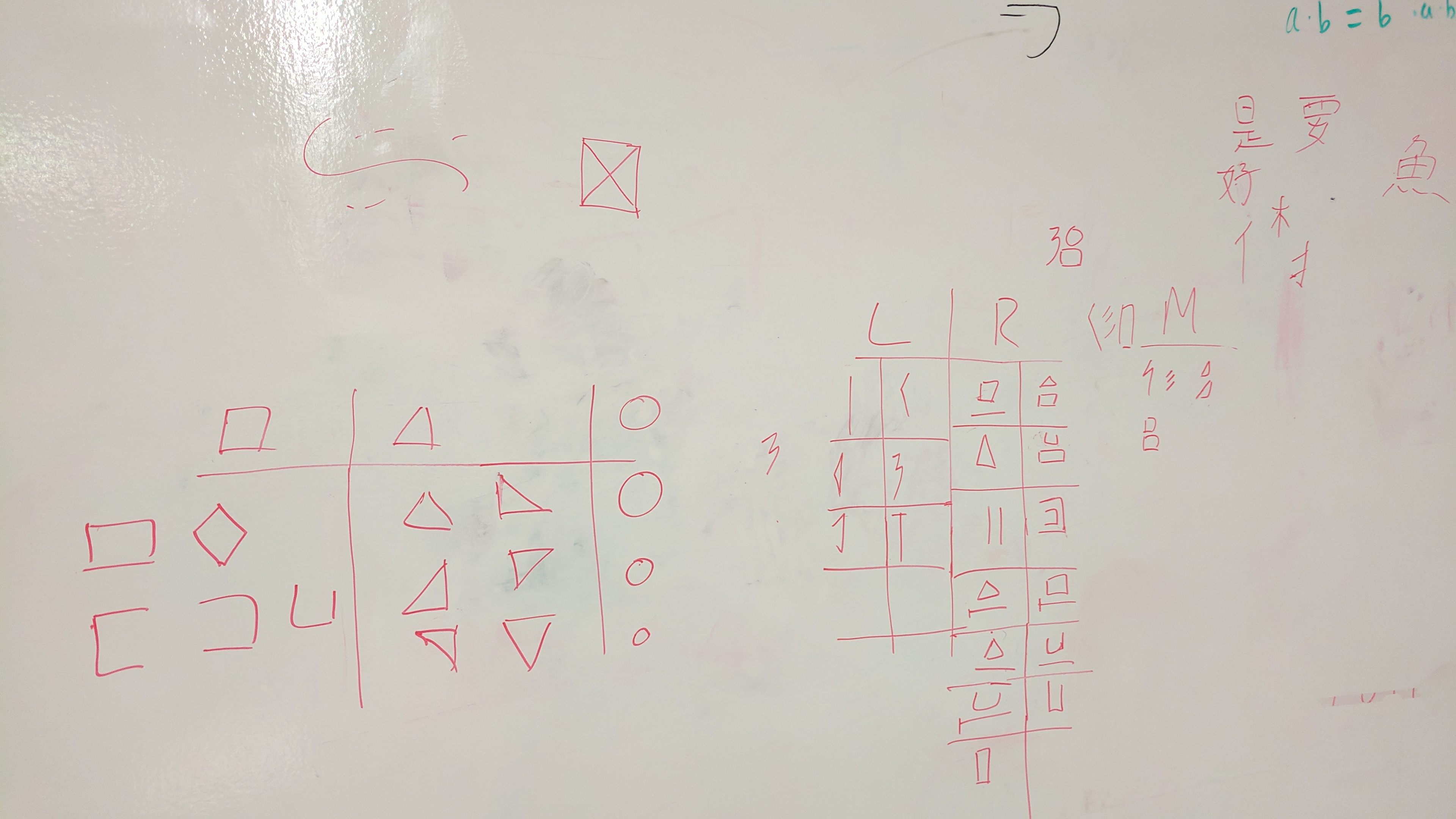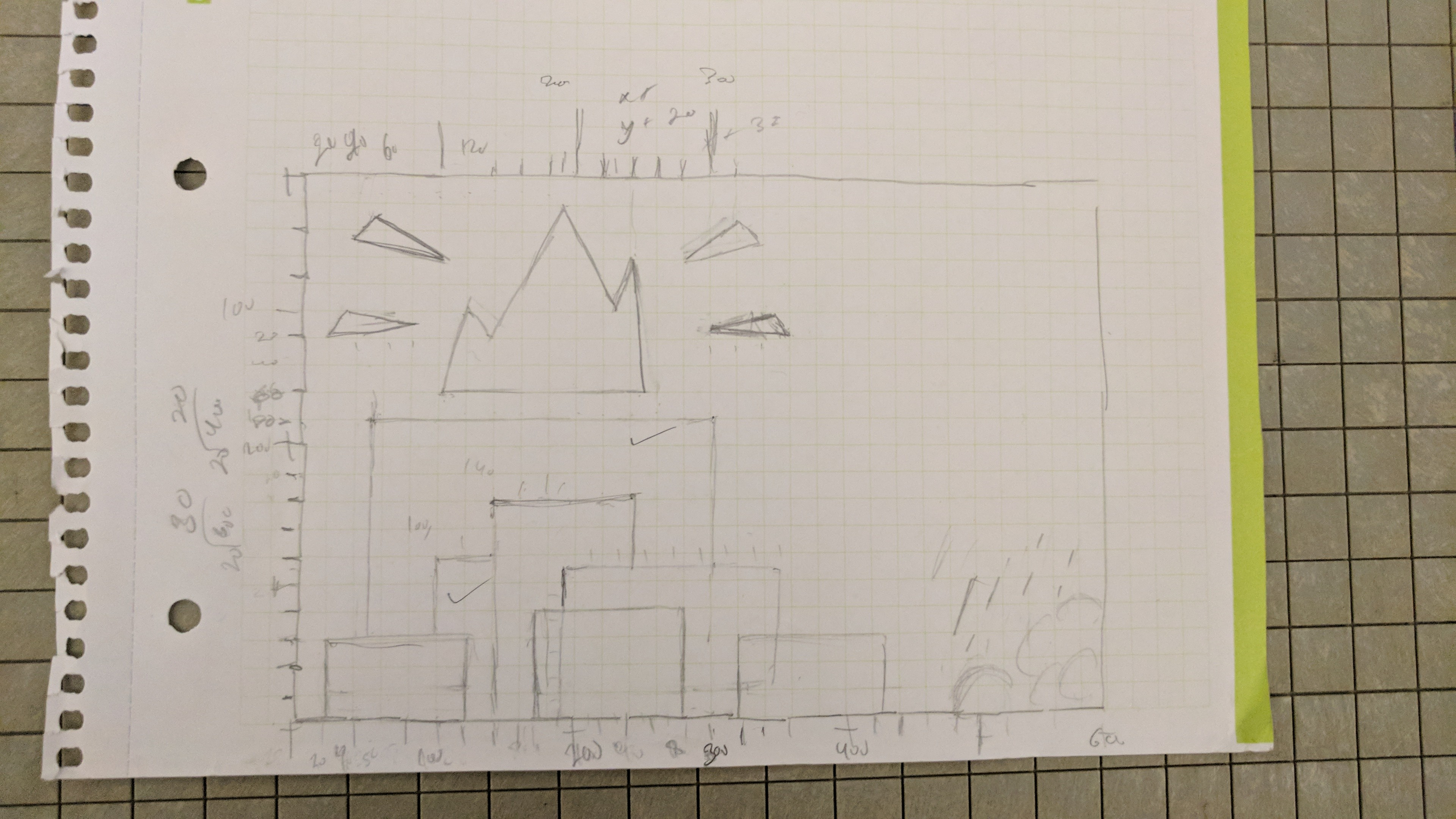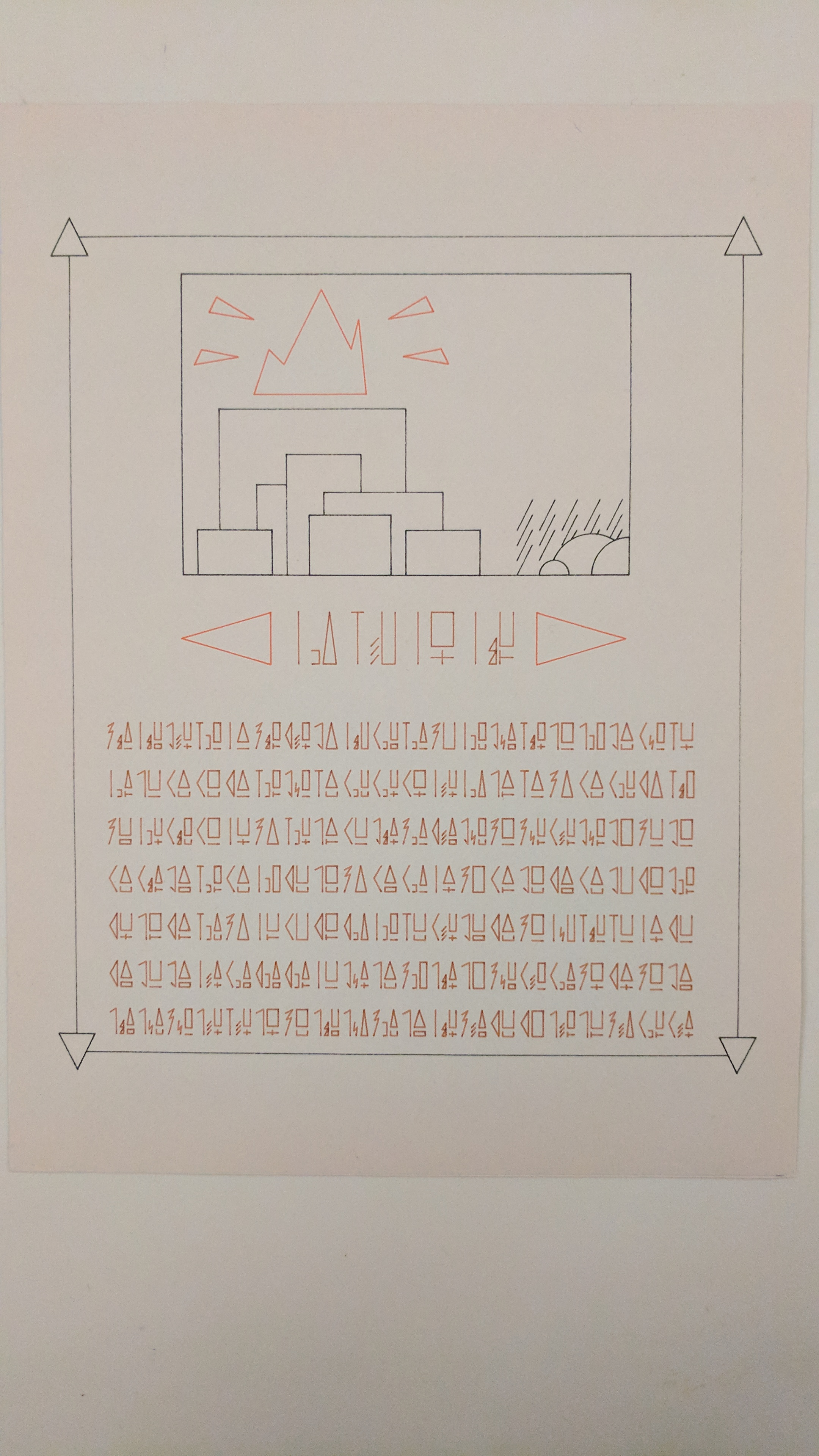# aahdee-Asemic

This was much more fun compared to the last time.

First up are my sketches. I’ve known basic to intermediate Chinese and took classes on it for years and I knew that for this project I wanted to take inspiration from that language. Below are my sketches.As shown, I took some common radicals from common characters and simplified them to get my radicals. Some of my radicals are original, but most are “borrowed” from Chinese. I also thought of the idea of an optional smaller middle radical. I got the inspiration from the Japanese Sokuon and the other small katakana that are used in the language.

I also wanted to make this look like a propaganda poster because of my liking to the government posters of the Cultural Revolution. Thus, I gave my work a bit of a orderly and proper feel to it, so it felt like an official document.

Here is a creation gif! Pleasing, isn’t it?The final shot:and the PDF:

aahdee_full_plotter

Now, a reflection. This project didn’t really feel too difficult for me, but it definitely was time consuming. I decided to approach this assignment in a modular way. There are two classes: Rad and Char for radicals and characters respectively. When every character is created, two or three radicals are also created with it. The radicals are randomly created and stored in an array for drawing later. Personally, I prefer using a plotter than a screen for display because I can ponder about the media used for delivery more. The type of paper, the colors, and the ink matters when making a piece with a plotter and not with a screen. There more room for experimentation and growth here.

Below is my code

```//the code for grabbing a pdf was gifted by Golan Levin and then modded slightly to create multiple pdfs by me import processing.pdf.*; boolean bRecordingPDF;   final int LRATIOX = 10; final int RRATIOX = 15; final int RATIOY = 35; final int RATIOX = 30; final int BUFFER = 5;   final int LRATIOXM = 8; final int MRATIOX = 5; final int RRATIOXM = 9; final int BUFFERM = 5;   final int LNUM = 7; final int MNUM = 5; final int RNUM = 17;   final int MARGIN = 100; final int SPACE = 10;     /*-----LEFT RADICALS-----*/ void drawVL(int x1, int y1, int x2, int y2) { int x = (x2+x1)/2; line(x, y1, x, y2); }   void drawLT(int x1, int y1, int x2, int y2) { int y = (y2+y1)/2; line(x2, y1, x1, y); line(x1, y, x2, y2); }   void drawTriL(int x1, int y1, int x2, int y2) { int y = (y2+y1)/2; triangle(x2, y1, x1, y, x2, y2); }   void drawM(int x1, int y1, int x2, int y2) { int ys = y1+((y2-y1)/7); int xm1 = x1+((x2-x1)/6); int ym1 = y1+(2*(y2-y1))/5; int xm2 = x1+(7*(x2-x1))/8; int ym2 = y1+((y2-y1)/3); line(x1, ys, x2, y1); line(x2, y1, xm1, ym1); line(xm1, ym1, xm2, ym2); line(xm2, ym2, x1, y2); }   void drawHook(int x1, int y1, int x2, int y2) { int ys = y1+((y2-y1)/7); line(x1, ys, x2, y1); line(x2, y1, x2, y2); }   void drawDHook(int x1, int y1, int x2, int y2) { int ys = y1+((y2-y1)/7); int xe = x1+((y2-y1)/8); int ye = y1+(5*(y2-y1)/6); line(x1, ys, x2, y1); line(x2, y1, x2, y2); line(x2, y2, xe, ye); }   void drawVT(int x1, int y1, int x2, int y2) { int xm = (x1+x2)/2; line(x1, y1, x2, y1); line(xm, y1, xm, y2); }   /*-----MIDDLE RADICALS-----*/   void drawLgtng(int x1, int y1, int x2, int y2) {   int y = y1+(y2-y1)/2; int xs = x1+((x2-x1)/4); int ym1 = y+(2*(y2-y)/3); int ym2 = y+(y2-y)/3; int xe = x1+(3*(x2-x1)/4); line(xs, y, x1, ym1); line(x1, ym1, x2, ym2); line(x2, ym2, xe, y2); }   void draw3L(int x1, int y1, int x2, int y2) { int y = y1+(y2-y1)/2; int ym1 = y+(y2-y)/3; int ym2 = y+2*(y2-y)/3; line(x2, y, x1, ym1); line(x2, ym1, x1, ym2); line(x2, ym2, x1, y2); }   void draw2T(int x1, int y1, int x2, int y2) { int y = y1+(y2-y1)/2; int ym = y+(y2-y)/2; triangle(x2, y, x2, ym, x1, ym); triangle(x2, ym, x2, y2, x1, y2); }   void drawSM(int x1, int y1, int x2, int y2) { int y = y1+(y2-y1)/2; int ys = y+(y2-y)/2; line(x1, ys, x2, ys); line(x2, ys, x2, y2); line(x2, y2, x1, y2); }   void drawLM(int x1, int y1, int x2, int y2) { int y = y1+(y2-y1)/2; line(x1, y, x2, y); line(x2, y, x2, y2); line(x2, y2, x1, y2); }   /*-----RIGHT RADICALS-----*/ //composed of square, triangles, u's, t's, and crosses   //special components   void drawCross(int x1, int y1, int x2, int y2) { int xm = (x1+x2)/2; int ym = (y1+y2)/2; line(xm, y1, xm, y2); line(x1, ym, x2, ym); } void drawU(int x1, int y1, int x2, int y2) { line(x1, y1, x1, y2); line(x1, y2, x2, y2); line(x2, y2, x2, y1); }   void drawHT(int x1, int y1, int x2, int y2) { int ym = (y1+y2)/2; line(x1, y1, x1, y2); line(x1, ym, x2, ym); }   //regular radicals void drawRec(int x1, int y1, int x2, int y2, String str) { if (str == "") { rect(x1, y1, x2-x1, y2-y1); } else { int yb = y1+2*(y2-y1)/3; int yr = yb+6; if (str == "l") { yb = y1+3*(y2-y1)/4; yr = yb+6; line(x1, y2, x2, y2); } else if (str == "u") { drawU(x1, yr, x2, y2); } else if (str == "t") { drawHT(x1, yr, x2, y2); } else if (str == "c") { drawCross(x1, yr, x2, y2); } rect(x1, y1, x2-x1, yb-y1); } }   void drawTri(int x1, int y1, int x2, int y2, String str) { int xm = (x1+x2)/2; if (str == "") { triangle(xm, y1, x1, y2, x2, y2); } else { int yt = y1+2*(y2-y1)/3; int yr = yt+6; if (str == "l") { yt = y1+3*(y2-y1)/4; line(x1, y2, x2, y2); } else if (str == "r") { rect(x1, yr, x2-x1, y2-yr); } else if (str == "u") { drawU(x1, yr, x2, y2); } else if (str == "t") { drawHT(x1, yr, x2, y2); } else if (str == "c") { drawCross(x1, yr, x2, y2); } triangle(xm, y1, x1, yt, x2, yt); } }   void drawLU(int x1, int y1, int x2, int y2, String str) { if (str == "") { drawU(x1, y1, x2, y2); } //wink wonk else { int yb = y1+2*(y2-y1)/3; int yr = yb+6; if (str == "l") { yb = y1+3*(y2-y1)/4; yr = yb+6; line(x1, y2, x2, y2); } else if (str == "r") { rect(x1, yr, x2-x1, y2-yr); } else if (str == "u") { drawU(x1, yr, x2, y2); } else if (str == "t") { drawHT(x1, yr, x2, y2); } else if (str == "c") { drawCross(x1, yr, x2, y2); } drawU(x1, y1, x2, yb); } }     //special boyes void draw2L(int x1, int y1, int x2, int y2) { line(x1, y1, x1, y2); line(x2, y1, x2, y2); }     void draw2U(int x1, int y1, int x2, int y2) { line(x1, y1, x2, y1); line(x2, y1, x2, y2); line(x2, y2, x1, y2);   int nx1 = x1+(x2-x1)/4; int nx2 = x1+2*(x2-x1)/3; int ny1 = y1+(y2-y1)/6; int ny2 = y1+5*(y2-y1)/6; line(nx1, ny1, nx2, ny1); line(nx2, ny1, nx2, ny2); line(nx2, ny2, nx1, ny2); }   //setup void drawLeft(int x1, int y1, int x2, int y2, int i) { if (i == 0) { drawVL(x1, y1, x2, y2); } else if (i == 1) { drawLT(x1, y1, x2, y2); } else if (i == 2) { drawTriL(x1, y1, x2, y2); } else if (i == 3) { drawM(x1, y1, x2, y2); } else if (i == 4) { drawHook(x1, y1, x2, y2); } else if (i == 5) { drawDHook(x1, y1, x2, y2); } else if (i == 6) { drawVT(x1, y1, x2, y2); } }   void drawMid(int x1, int y1, int x2, int y2, int i) { if (i == 0) { drawLgtng(x1, y1, x2, y2); } else if (i == 1) { draw3L(x1, y1, x2, y2); } else if (i == 2) { draw2T(x1, y1, x2, y2); } else if (i == 3) { drawSM(x1, y1, x2, y2); } else if (i == 4) { drawLM(x1, y1, x2, y2); } }   void drawRig(int x1, int y1, int x2, int y2, int i) { String[] tag = {"", "l", "u", "t", "c", "r"}; if (0 &lt;= i &amp; i &lt;= 4) //rect doesnt have the option r { String t = tag[i]; drawRec(x1, y1, x2, y2, t); } else if (5 &lt;= i &amp;&amp; i &lt;= 10) { String t = tag[i-5]; drawTri(x1, y1, x2, y2, t); } else if (11 &lt;= i &amp;&amp; i &lt;= 16) { String t = tag[i-11]; drawLU(x1, y1, x2, y2, t); } else if (i == 3) { draw2L(x1, y1, x2, y2); } else if (i == 4) { draw2U(x1, y1, x2, y2); } } class Rad { String type; //coordinates are the top left and bottom right int x1, y1, x2, y2, index, scale; public Rad(int x1c, int y1c, int scale, String tc) { x1 = x1c; y1 = y1c; type = tc; if (type == "L") { x2 = x1+(LRATIOX*scale); y2 = y1+(RATIOY*scale); index = int(random(LNUM)); } else if (type == "Lm") { x2 = x1+(LRATIOXM*scale); y2 = y1+(RATIOY*scale); index = int(random(LNUM)); } else if (type == "M") { x2 = x1+(MRATIOX*scale); y2 = y1+(RATIOY*scale); index = int(random(MNUM)); } else if (type == "R") { x2 = x1+(RRATIOX*scale); y2 = y1+(RATIOY*scale); index = int(random(RNUM)); } else if (type == "Rm") { x2 = x1+(RRATIOXM*scale); y2 = y1+(RATIOY*scale); index = int(random(RNUM)); } } void drawRad() { if (type == "L" || type == "Lm") { drawLeft(x1, y1, x2, y2, index); } if (type == "M") { drawMid(x1, y1, x2, y2, index); } if (type == "R" || type == "Rm") { drawRig(x1, y1, x2, y2, index); } } } class Char { int x, y, scaleoff; boolean mbool; Rad left, middle, right; public Char(int xc, int yc, int scale) { x = xc; y = yc; int bool = int(random(2)); scaleoff = (scale &gt; 1 ? 4*scale : 0); mbool = (bool == 0 ? true : false); if (mbool) { left = new Rad(x, y, scale, "Lm"); middle = new Rad(x+LRATIOXM+(scale*BUFFERM+scaleoff), y, scale, "M"); right = new Rad(x+LRATIOXM+(scale*(2*BUFFERM)+scaleoff)+MRATIOX, y, scale, "Rm"); } else { left = new Rad(x, y, scale, "L"); right = new Rad(x+LRATIOX+(scale*BUFFER+scaleoff), y, scale, "R"); } }   public void drawChar() { left.drawRad(); right.drawRad(); if (mbool) { middle.drawRad(); } } }   void drawImage(int x, int y) { //this erases the previous draw. this is done to fix some overlap issues with the circles and the frame rect(x, y, 600, 400);   //pedestal line(x+50, y+180, x+50, y+340); line(x+50, y+180, x+300, y+180); line(x+300, y+180, x+300, y+290);   line(x+100, y+280, x+100, y+340); line(x+100, y+280, x+140, y+280);   line(x+140, y+240, x+140, y+400); line(x+140, y+240, x+240, y+240); line(x+240, y+240, x+240, y+290);   line(x+190, y+290, x+190, y+320); line(x+190, y+290, x+350, y+290); line(x+350, y+290, x+350, y+340);   rect(x+170, y+320, 110, 80); rect(x+20, y+340, 100, 60); rect(x+300, y+340, 100, 60);   //shadows line(x+470, y+300, x+450, y+340); line(x+470, y+320, x+450, y+360); line(x+470, y+340, x+450, y+380); line(x+470, y+360, x+450, y+400);   line(x+500, y+300, x+480, y+340); line(x+500, y+320, x+480, y+360); line(x+500, y+340, x+480, y+380);   line(x+530, y+300, x+510, y+340); line(x+530, y+320, x+510, y+360); line(x+530, y+340, x+523, y+352);   line(x+560, y+300, x+540, y+340); line(x+560, y+320, x+548, y+345); line(x+560, y+340, x+557, y+346);   line(x+590, y+300, x+570, y+340); line(x+590, y+320, x+574, y+351); line(x+590, y+340, x+583, y+353);   //circles arc(x+550, y+400, 110, 110, PI+.4, TWO_PI-QUARTER_PI-.2); arc(x+600, y+400, 100, 100, PI, TWO_PI-2*QUARTER_PI); arc(x+500, y+400, 40, 40, PI, TWO_PI);   //frame noFill(); rect(x, y, 600, 400); }   void drawIdol(int x, int y) { //idol line(x+100, y+160, x+250, y+160); line(x+100, y+160, x+120, y+100); line(x+120, y+100, x+140, y+120); line(x+140, y+120, x+190, y+20); line(x+190, y+20, x+230, y+100); line(x+230, y+100, x+240, y+60); line(x+240, y+60, x+250, y+160);   //triangles triangle(x+20, y+120, x+30, y+100, x+80, y+110); triangle(x+40, y+50, x+50, y+30, x+100, y+60); triangle(x+340, y+50, x+330, y+30, x+280, y+60); triangle(x+300, y+110, x+350, y+100, x+360, y+120); }     void drawBookends() { triangle(320, 550, 320, 620, 200, 585); triangle(680, 550, 680, 620, 800, 585); }   void drawBorder() { line(MARGIN/2+13, MARGIN/2, width-(MARGIN/2)-13, MARGIN/2); line(width-(MARGIN/2), MARGIN/2+25, width-(MARGIN/2), height-(MARGIN/2)-25); line(MARGIN/2+13, height-MARGIN/2, width-(MARGIN/2)-13, height-MARGIN/2); line((MARGIN/2), MARGIN/2+25,(MARGIN/2), height-(MARGIN/2)-25);   triangle(MARGIN/2, MARGIN/4, MARGIN/4, (3*MARGIN)/4, (3*MARGIN)/4, (3*MARGIN)/4); triangle(width-MARGIN/2, MARGIN/4, width-MARGIN/4, (3*MARGIN)/4, width-(3*MARGIN)/4, (3*MARGIN)/4); triangle(MARGIN/2, height-MARGIN/4, MARGIN/4, height-(3*MARGIN)/4, (3*MARGIN)/4, height-(3*MARGIN)/4); triangle(width-MARGIN/2, height-MARGIN/4, width-MARGIN/4, height-(3*MARGIN)/4, width-(3*MARGIN)/4, height-(3*MARGIN)/4); }   Char[] cList = new Char; Char[] title = new Char;   void setup() { size(1000, 1200); background(255); int index = 0; for (int i = 0; i &lt; title.length; i++) { title[i] = new Char((i*(RATIOX*2+20))+350, 550, 2); }   for (int i = MARGIN; i &lt;= width-MARGIN; i+= (RATIOX+SPACE)) { for (int j = 700; j &lt;= height-MARGIN; j+= (RATIOY+SPACE)+20) { if (index &lt; cList.length) { Char c = new Char(i, j, 1); cList[index] = c; } index++; } } }   void keyPressed() { // When you press a key, it will initiate a PDF export bRecordingPDF = true; }   void draw() { strokeWeight(2); noFill(); drawBorder(); drawImage(200, 100); drawIdol(200, 100); drawBookends(); for (int i = 0; i &lt; title.length; i++) { title[i].drawChar(); } for (int i = 0; i &lt; cList.length; i++) { cList[i].drawChar(); } if (bRecordingPDF) { background(255); noFill(); beginRecord(PDF, "aahdee_black.pdf"); drawBorder(); drawImage(200, 100); endRecord();   background(255); noFill(); beginRecord(PDF, "aahdee_red.pdf"); drawIdol(200, 100); drawBookends(); endRecord();   background(255); noFill(); beginRecord(PDF, "aahdee_brown.pdf"); for (int i = 0; i &lt; title.length; i++) { title[i].drawChar(); } for (int i = 0; i &lt; cList.length; i++) { cList[i].drawChar(); } endRecord();   background(255); noFill(); beginRecord(PDF, "aahdee_full.pdf"); drawBorder(); drawImage(200, 100); drawIdol(200, 100); drawBookends(); for (int i = 0; i &lt; title.length; i++) { title[i].drawChar(); } for (int i = 0; i &lt; cList.length; i++) { cList[i].drawChar(); } endRecord(); bRecordingPDF = false; } }```